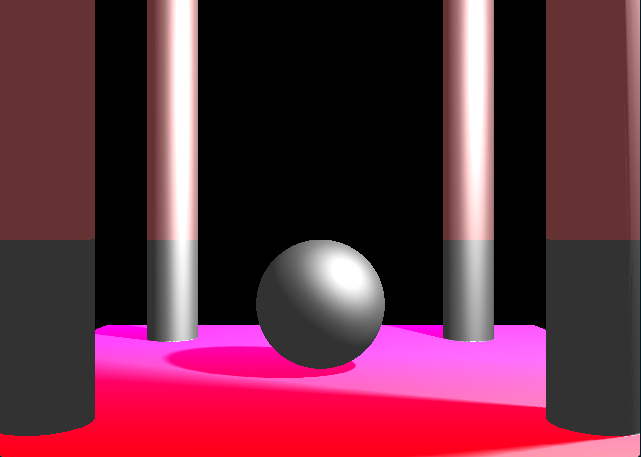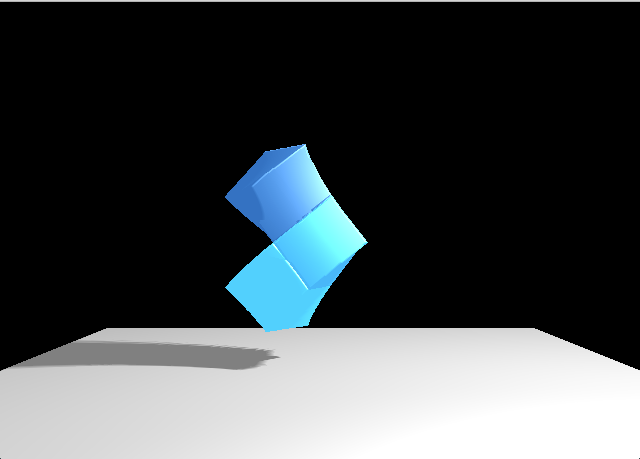# Ray Marching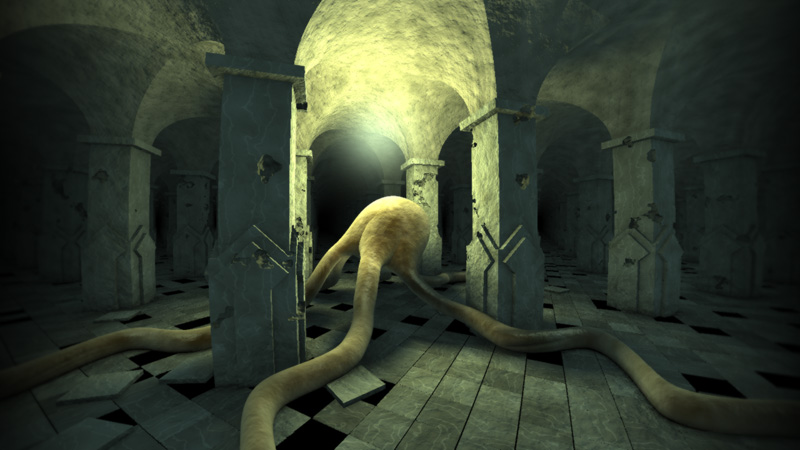A ShiftForward tech talk by João Costa / @JD557

• Based on the work of Iñigo Quílez (iq/rgba)
• Code examples in Scala (CPU)
• Practical application should be implemented for GPUs

## Overview

• Quick Rendering Intro
• A Simple Ray Tracer
• Distance Fields
• Distance Field Operations
• Illumination

## Quick Rendering Intro

• Rasterization
• Ray Tracing*
• Ray Casting
• Path Tracing
• Ray Marching
• ...

*Some ray tracing methods go by multiple names and some names apply to multiple methods. The following definitions might not be accurate.

## Rasterization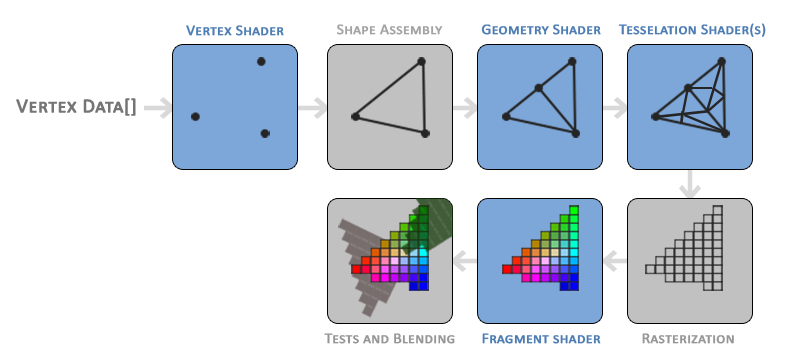## Ray Casting

• Simplest form of ray tracing
• Cast a ray for each pixel (or vertical scanline) of the image plane
• Solve ray-surface intersection test
•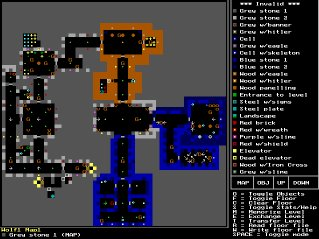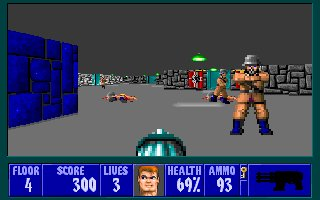## Path tracing

• Solve the rendering equation via Monte-Carlo integration
• Trace a rays with weighted random reflections/transmissions
• Multiple iterations are required to avoid noisy images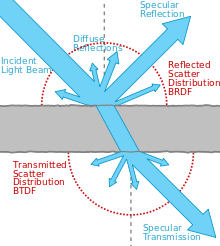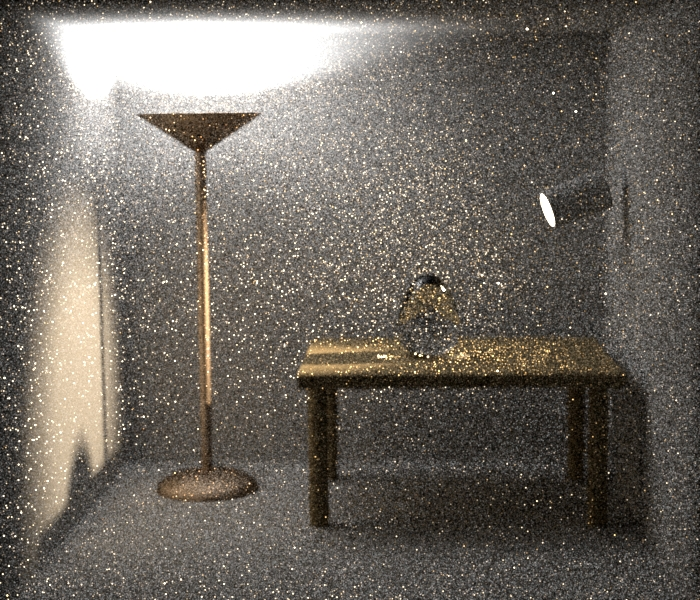## Ray marching

• Also known as sphere marching
• Objects and scenes are represented as distance fields
• Resulting image is calculated by marching through the distance field

## A simple ray tracer

Object: collision function $f:R^3 \rightarrow Boolean$

eg.: $sphere(x, y, z) = \sqrt{x^2 + y^2 + z^2} \leq 1$


case class Vec3d(x: Double, y: Double, z: Double) { ... }
type Object3d = Vec3d => Boolean
val sphere: Object3d =
(v: Vec3d) => sqrt(v.x*v.x + v.y*v.y + v.z*v.z) <= 1.0

• Trace a ray from the eye to each pixel of the image plane
• "March" through it in small $\vec \Delta$ of size $\epsilon$
• If it hits someting, render a color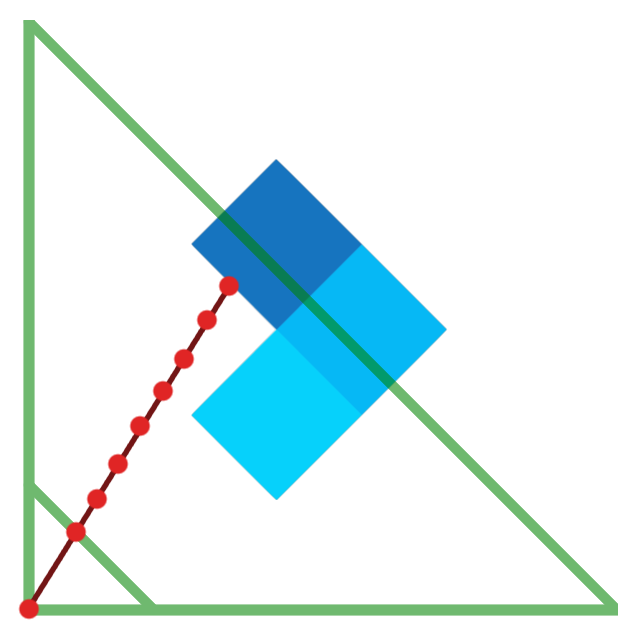Note: this is not ray marching


def traceRay(x: Double, y: Double, obj: Object3d, eps: Double) = {
val initVector = Vec3d(x, y, zNear)
val delta = initVector.normalize
def traceRayAux(pos: Vec3d): Color = obj(pos) match {
case true =>
Color(255, 255, 255)
case false =>
if (pos.z > zFar) Color(0, 0, 0)
else traceRayAux(pos + delta*eps)
}
traceRayAux(initVector)
}


### Colors and textures

Colors/3d textures can be easilly implemented:


type Object3d = Vec3d => Option[Color]


This will be ommited in this talk, as it adds unnecessary complexity to most examples.

### Limitations

• Small $\epsilon$:
• Slow to converge
• Large $\epsilon$:
• Small objects can be skipped
• Objects will have "dents"

## Distance fields

Object: signed distance function of a point to its surface $sdf:R^3 \rightarrow R$

eg.: $sphere(x, y, z) = \sqrt{x^2 + y^2 + z^2} - 1$


case class Vec3d(x: Double, y: Double, z: Double) { ... }
type DistanceField = Vec3d => Double
val sphere: DistanceField =
(v: Vec3d) => sqrt(v.x*v.x + v.y*v.y + v.z*v.z) - 1.0• $sdf(\vec p)$ is the distance to the closest object to $\vec p$
• Therefore, $sdf(\vec p)$ is always a safe value for $\left | \vec\Delta \right |$
• In practice, the $\left | \vec\Delta \right | = max(sdf(p), \epsilon)$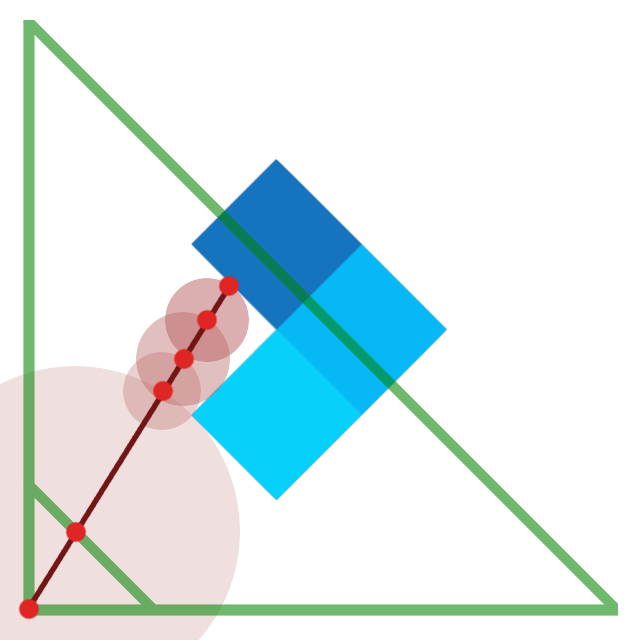def traceRay(x: Double, y: Double, sdf: DistanceField, eps: Double) = {
val initVector = Vec3d(x, y, zNear)
val delta = initVector.normalize
def traceRayAux(pos: Vec3d): Color = sdf(pos) match {
case dist if dist <= 0 =>
Color(255, 255, 255)
case dist =>
if (pos.z > zFar) Color(0, 0, 0)
else traceRayAux(pos + delta*max(dist, eps))
}
traceRayAux(initVector)
}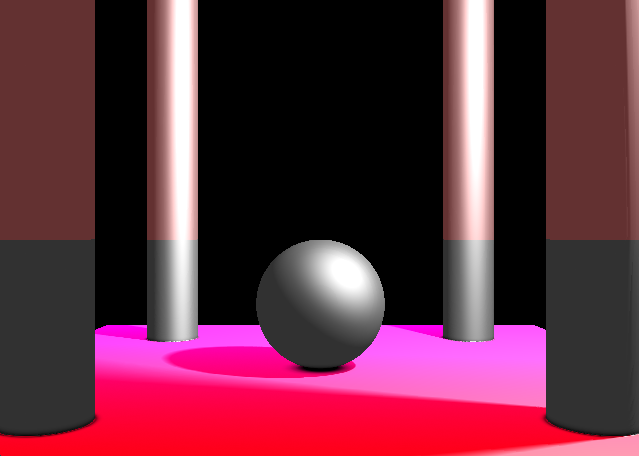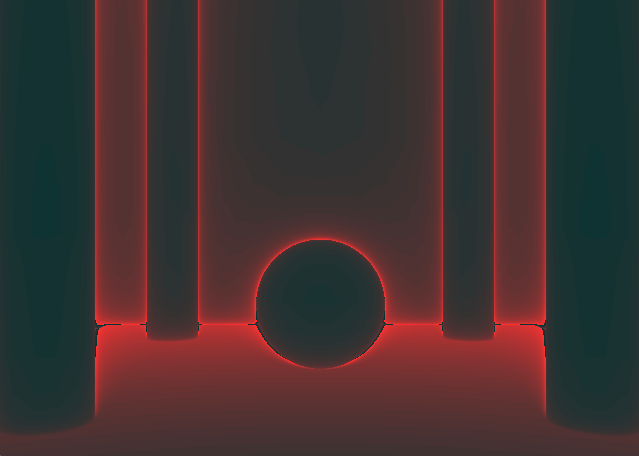$Color(r,g,b) = (iterations \times 5, 50, 50)$

## Distance Field Operations

• By manipulating distance fields, we can implement:
• Boolean operations
• Geometric transformations
• Crazy stuff

### Boolean Operations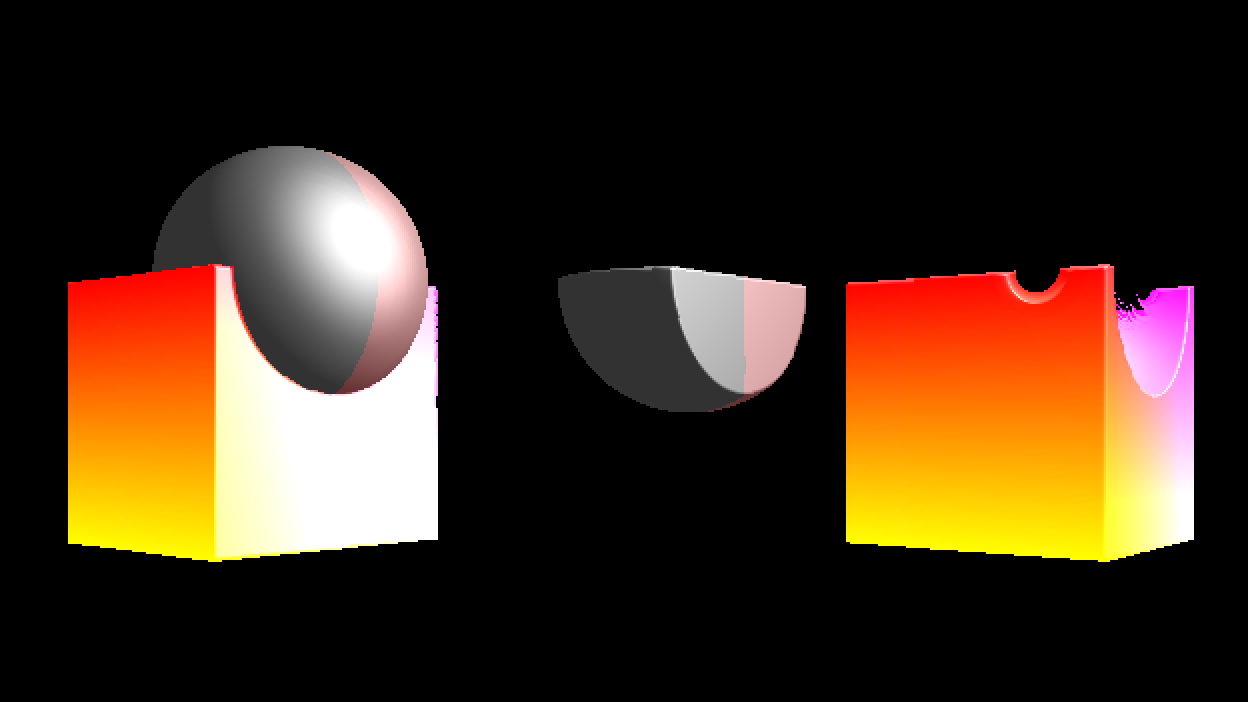### Boolean Operations

#### Union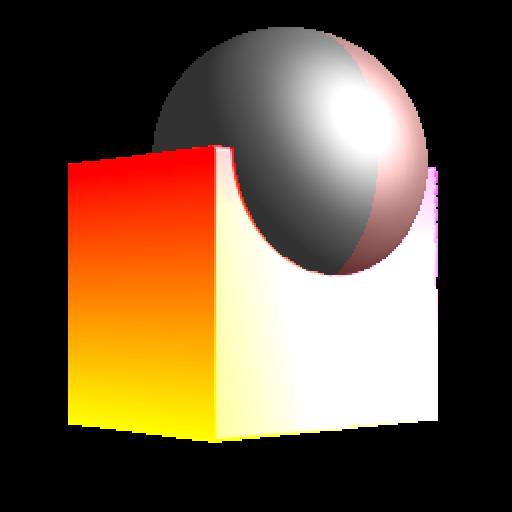$$obj_1 \cup obj_2 = min(sdf_1(\vec p), sdf_2(\vec p))$$

### Boolean Operations

#### Intersection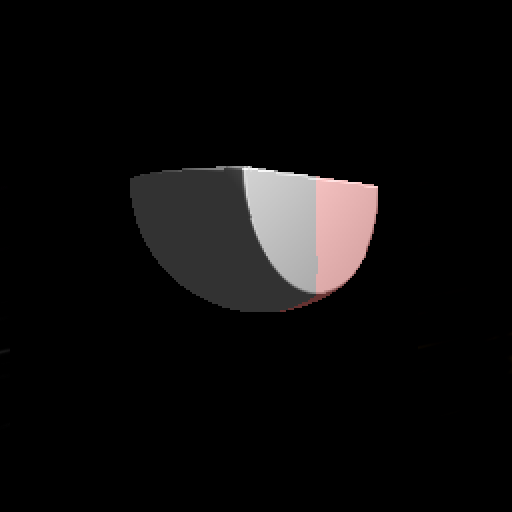$$obj_1 \cap obj_2 = max(sdf_1(\vec p), sdf_2(\vec p))$$

### Boolean Operations

#### Difference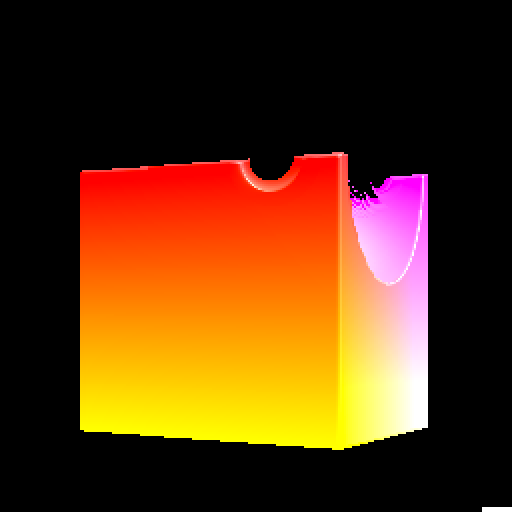$$obj_1 - obj_2 = obj_1 \cap (\neg obj_2) = max(sdf_1(\vec p), -sdf_2(\vec p))$$

• Translation
• Rotation
• Scale

### Geometric Transformations

#### Translation/Rotation

• Moving objects is hard
• Moving our ray is easy
• Apply $T$ to objects $=$ Apply $T^{-1}$ to rays

### Geometric Transformations

#### Translation/Rotation


def translate(delta: Vec3d)(sdf: DistanceField): DistanceField =
(v: Vec3d) => sdf(v - delta)

def rotateX(theta: Double)(sdf: DistanceField): DistanceField =
(v: Vec3d) => sdf(v.rotX(-theta))

def rotateY(theta: Double)(sdf: DistanceField): DistanceField =
(v: Vec3d) => sdf(v.rotY(-theta))

def rotateZ(theta: Double)(sdf: DistanceField): DistanceField =
(v: Vec3d) => sdf(v.rotZ(-theta))


### Geometric Transformations

#### Scaling

• The same trick applies to scaling
• However, scaling a distance field will also scale our distances
• Rescale the returned distance to know how much to march

### Geometric Transformations

#### Scaling


def scale(s: Double)(sdf: DistanceField): DistanceField =
(v: Vec3d) => sdf(v / s) * s


### Crazy stuff

• We assumed that $sdf$ is continuous and exact
• We can break the rules (slightly) to obtain cool effects:
• Modulo operations: Infinite repetition
• Rotations with variable $\theta$: Twist
• Union with smooth min: Blend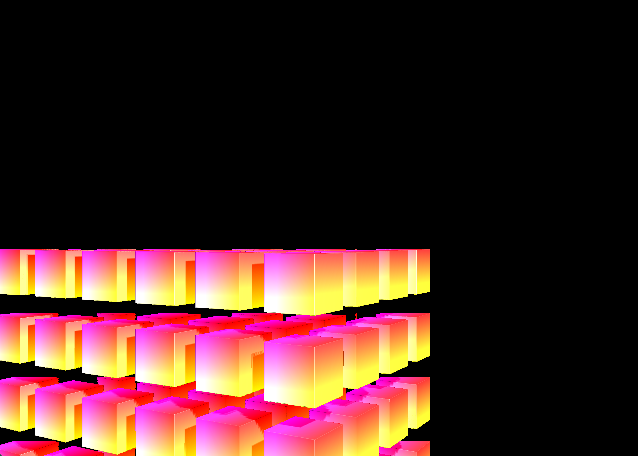## Illumination

A simple illumination model: $$ambient + \sum_L shadow_L (diffuse_L + specular_L)$$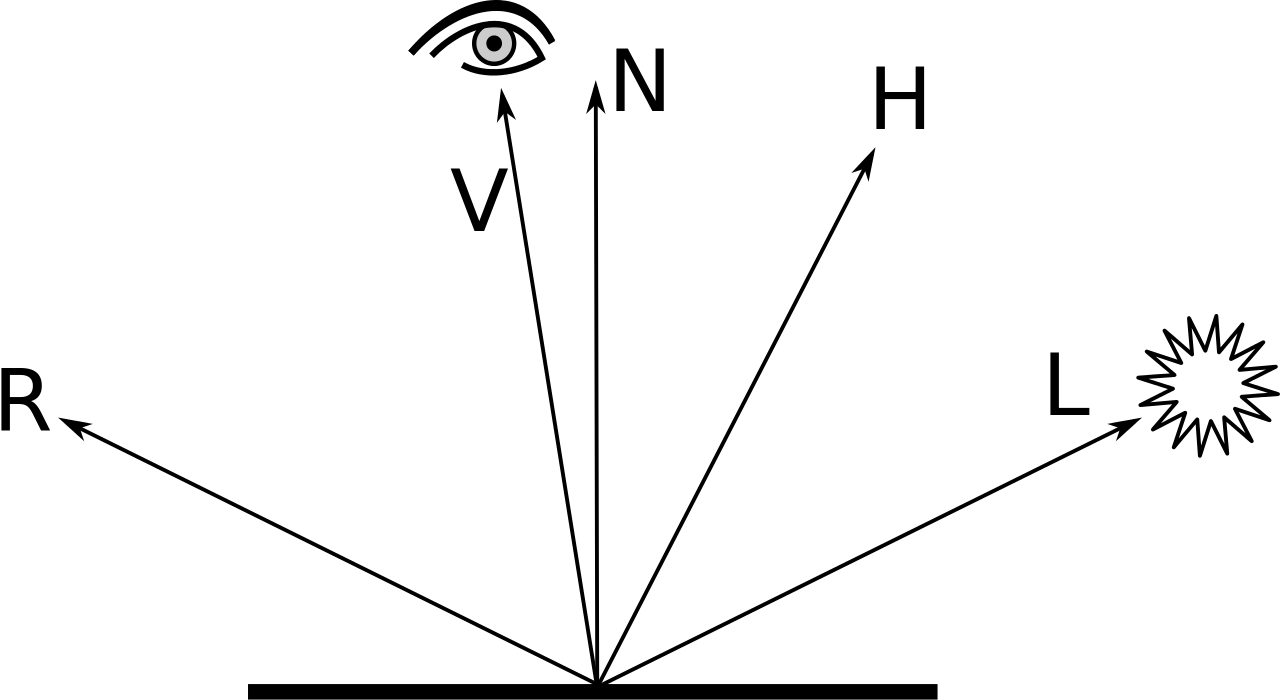• Global:
• $ambient = occlusion \times K_{ambient}$
• For each light $L$:
• $shadow_L$
• $diffuse_L = L_{diffuse} \times K_{diffuse} \times max(\vec{N} \cdot \vec{L}, 0)$
• $specular_L = L_{specular} \times K_{specular} \times max(\vec{R} \cdot \vec{V}, 0)^\alpha$
• How to calculate the normal vector
• How to calculate the ambient occlusion
• How to calculate shadowed areas

### Normals

$\vec{N} = \nabla sdf = \left (\frac{\partial sdf}{\partial x}, \frac{\partial sdf}{\partial y}, \frac{\partial sdf}{\partial z} \right )$

def normal(p: Vec3d, scene: DistanceField, eps: Double): Vec3d = {
val dx = scene(p + (eps, 0, 0)) - scene(p - (eps, 0, 0))
val dy = scene(p + (0, eps, 0)) - scene(p - (0, eps, 0))
val dz = scene(p + (0, 0, eps)) - scene(p - (0, 0, eps))
new Vec3d(dx, dy, dz).normalized
}


### Ambient Occlusion

• Constant ambient light: naïve appoximation to global illumination
• Intuition:
• Open areas should be well lit
• Small confined spaces should be dark
• Idea:
• If an object is on an open area: $sdf(\vec p + \vec N \times \epsilon) \approx \epsilon$
• If an object is on an closed area: $sdf(\vec p + \vec N \times \epsilon) \approx 0$

### Ambient Occlusion

• Solution: for a point $\vec p$:
• Probe $N$ (eg. 6) points $\vec{p'_i} = \vec p + (\vec N \times \epsilon \times i)$
• Combine the real distance and the expected distance with a "magic formula", eg.: $$occlusion = 1 - \frac{\sum_i^N 2^{-i}(\epsilon \times i - sdf(p'_i))}{\sum_i^N 2^{-i} (\epsilon \times i)}$$

### Ambient Occlusion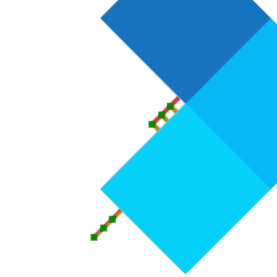### Ambient Occlusion


def ambientOcclusion(p: Vec3d, n: Vec3d, scene: DistanceField,
epsilon: Double, iter: Int) = {
val bias = scene(p)
def occlusionAux(i: Int, accum: Double, total: Double): Double =
i match {
case 0 => accum / total
case i =>
val expected = bias + (i * epsilon)
val real = scene(p + n * (i * epsilon))
val decay = pow(2, i)
occlusionAux(i-1,
accum+(expected-real)/decay, total+expected/decay)
}
1 - occlusionAux(iter, 0, 0)
}


### Ambient Occlusion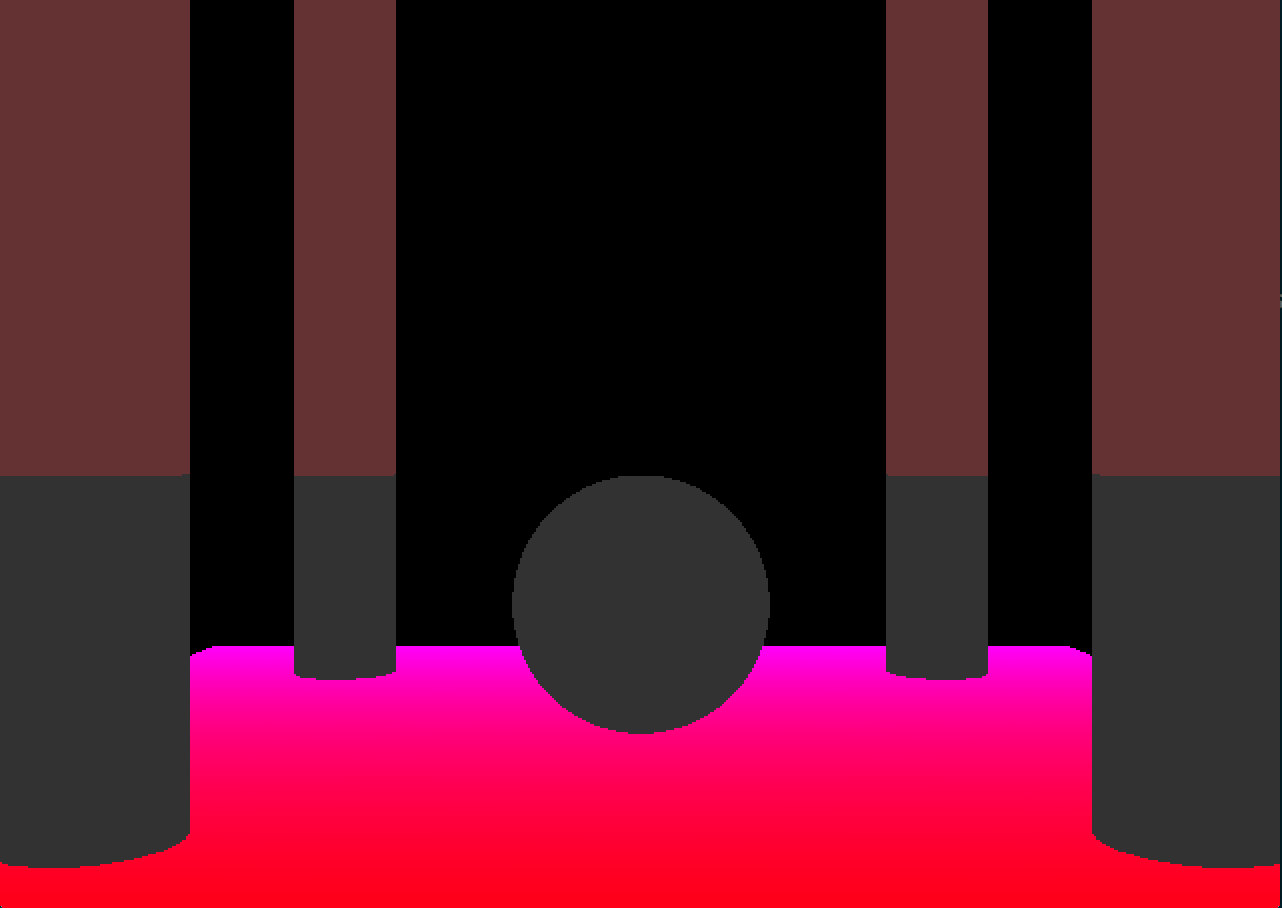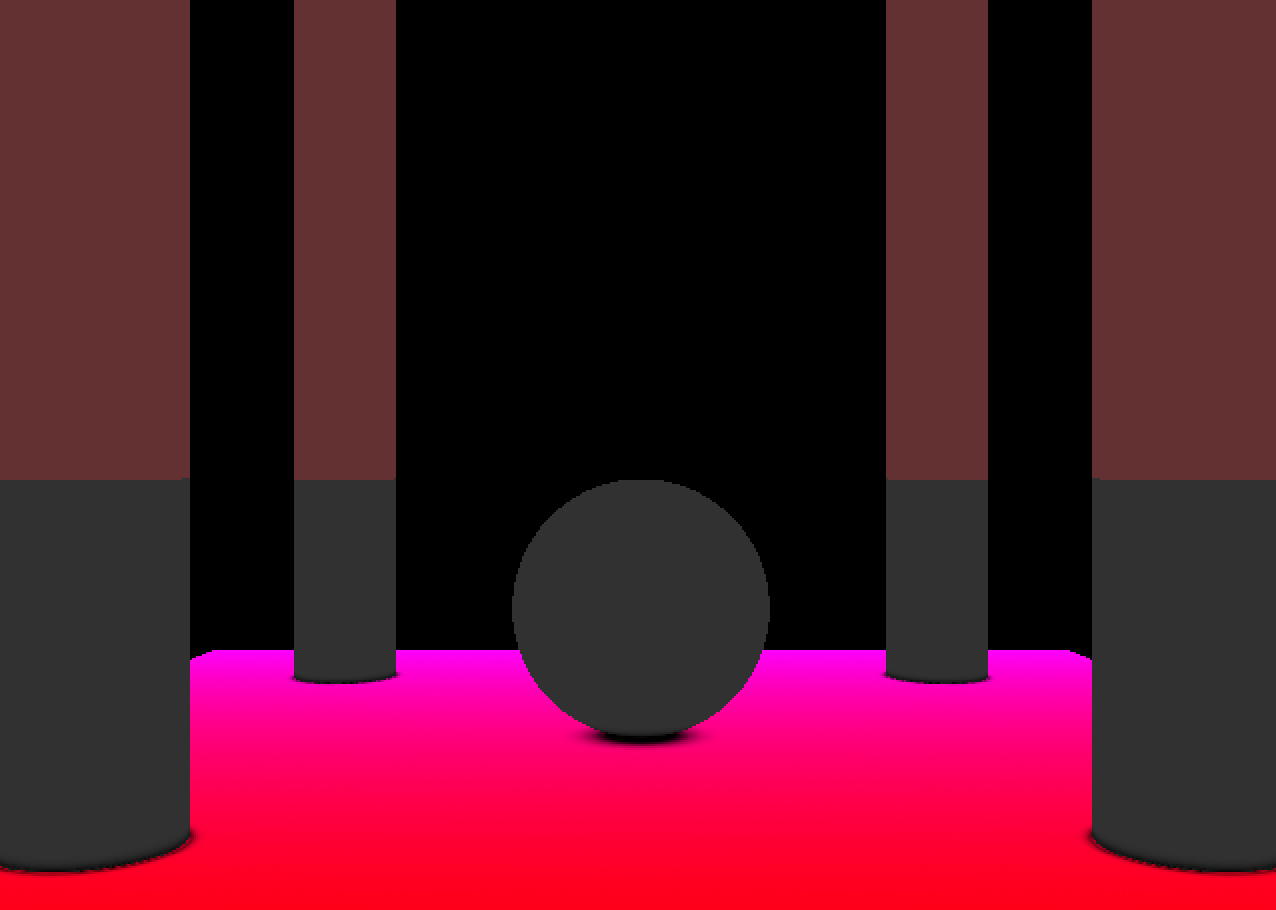• Simple: Send shadow feelers using ray marching!
• Trace a ray from the object towards the light
• If it hits something, it's shadowed
• If it reaches the light, it's not

• Soft-shadows: combine the $N$ distances with a "magic formula"
• The heuristic should be disabled on the first few points:
• Avoids artifacts (the ray will start close to the object)
• We still need to consider collisions
• Shadows should get smoother with the distance


def shadow(p: Vec3d, lPos: Vec3d, sdf: DistanceField, eps: Double, k: Int) = {
val init = (lPos - p).normalized
def shadowAux(delta: Vec3d, acc: Double): Double =
if (delta.size > (lPos - p).size) acc
else
if (sdf(p + delta) <= 0.0) 0.0
else if (delta.size * k <= 1.0)
shadowAux(delta + init * max(sdf(p + delta), eps), acc)
else
shadowAux(delta + init * max(sdf(p + delta), eps),
min(acc, k * dist / delta.size))
}

K is a constant to define how smooth how shadows will be. The smaller the k, the smoother the shadows. 32 is a nice value.